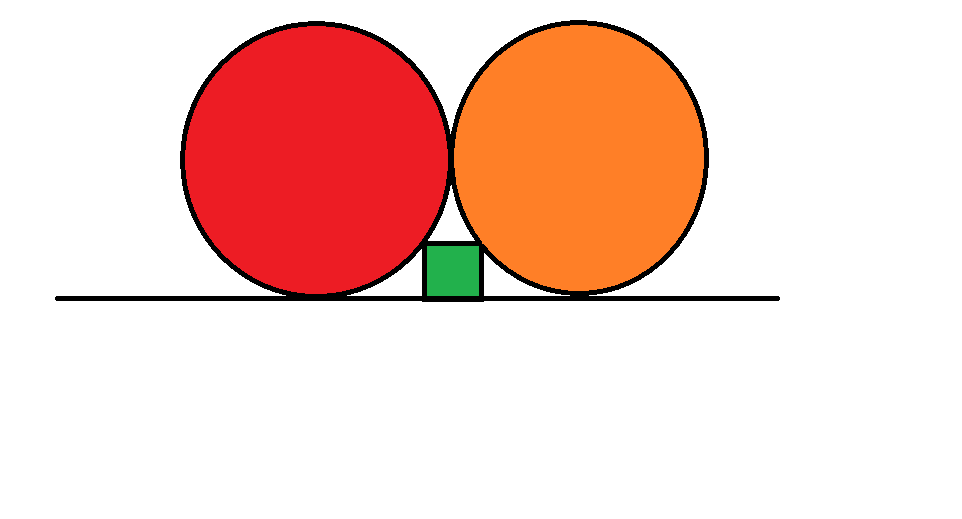# Two Circles and a Square

Geometry Level 3Two identical touching circles of radius $r$ share the same tangent. A square of side $s$, rests on the tangent and touches the two circles as shown in the diagram above.

If $r$ is 10 units. Then the side $s$ of the square is how many units?

×